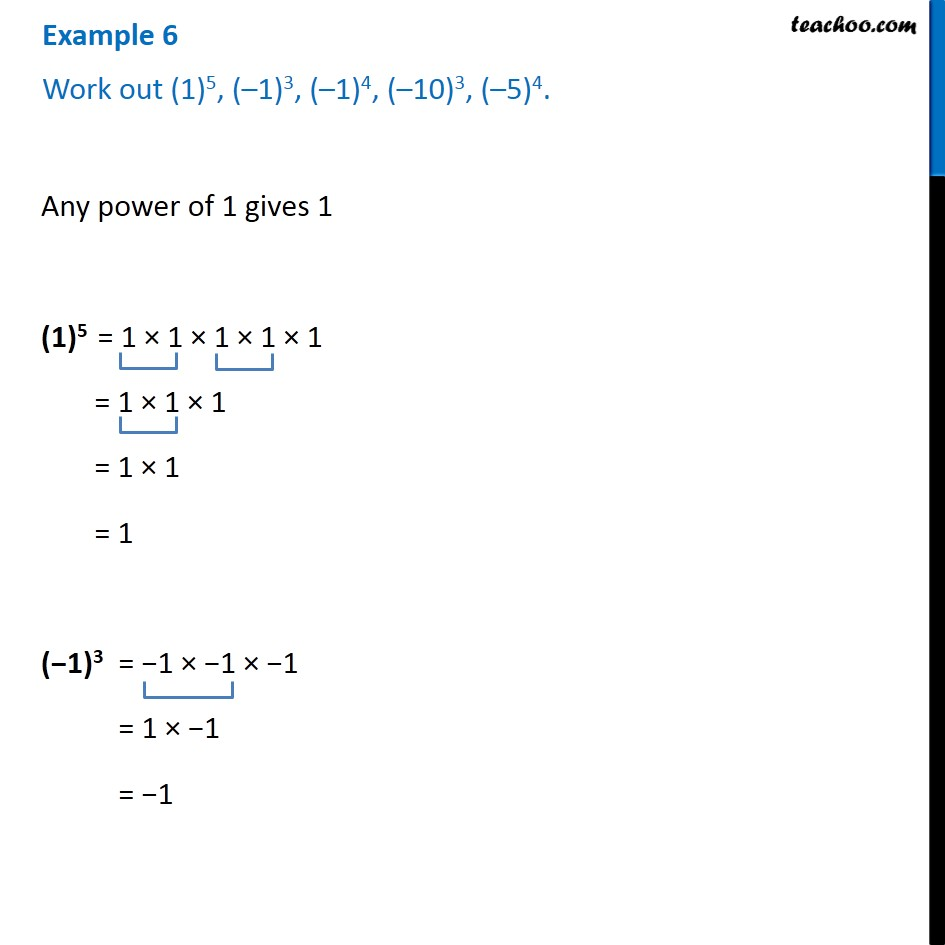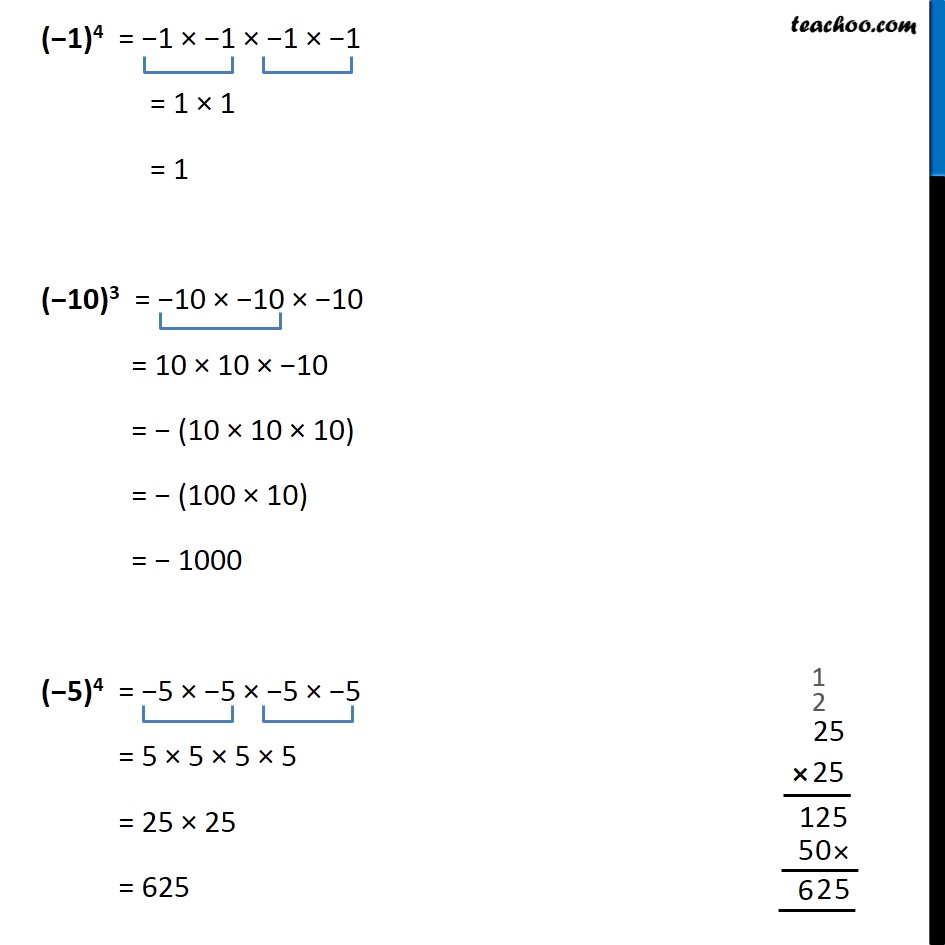1. Chapter 13 Class 7 Exponents and Powers
2. Concept wise
3. Power of 1 and -1

Transcript

Example 6 Work out (1)5, (–1)3, (–1)4, (–10)3, (–5)4. Any power of 1 gives 1 (1)5 = 1 × 1 × 1 × 1 × 1 = 1 × 1 × 1 = 1 × 1 = 1 (−1)3 = −1 × −1 × −1 = 1 × −1 = −1 (−1)4 = −1 × −1 × −1 × −1 = 1 × 1 = 1 (−10)3 = −10 × −10 × −10 = 10 × 10 × −10 = − (10 × 10 × 10) = − (100 × 10) = − 1000 (−5)4 = −5 × −5 × −5 × −5 = 5 × 5 × 5 × 5 = 25 × 25 = 625

Power of 1 and -1